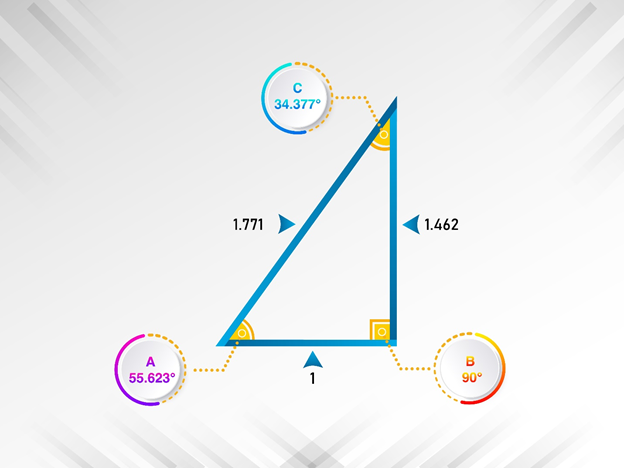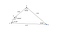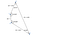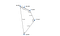# Dancing with Phigons

by Jim FonsecaFigure 1: The Golden Right Triangle

You’ve heard of the Golden Ratio, that magical, mystical number 1.61803…, like pi, an endless, non-repeating number called phi, symbolized by Φ. Two lines cut in that ratio have given us Golden Triangles, Golden Rectangles and Golden Gnomons and a few others, a cluster of polygons that I propose we call phigons. (Not to be confused with the musical group or science fiction character of that name, lol.) And you may also know that phi can be found in everything from the diagonals of a pentagon to the ratio of successive numbers in the Fibonacci sequence. If you want a brief review of how Φ is derived, I’ve added that at the end of this article.

All these Golden figures (phigons) are based on the length of sides. But wait — did you hear that splat? — the dancer trying to create a triangle with lengths in Φ proportion fell flat on the dance floor. Three lengths in Φ proportion, say, 1.0, 1,618 and 2.618, are too short to meet the basic criterion of forming a triangle — any two sides must exceed the length of the third side. All the existing Golden figures are based on phi relationship between only two sides of the triangles.

So I propose a new type of Golden Triangle based on its angles - Figure 1. After all, although it is sometimes called a trilateral or a trigon, in common parlance we call it a triangle. It’s shown above in Figure 1 and it contains a big surprise — it’s a right triangle! I’ll show you the formula below. I’ve jokingly called it the Fonseca Triangle, but a more appropriate name is the Golden Right Triangle.

Given that the angles of a triangle sum to 180°, based on how we calculate phi, the formula to solve for three angles in Φ proportion becomes

This simplifies to

When we solve the formula, x is precisely 90°. The other two angles are approximately 55.623° (angle A) and 34.377° (angle C). Some folks will wonder if this is the same golden triangle known as the Kepler triangle, which is also a right triangle but based on sides of 1, Φ and the square root of Φ. But it’s not the same — the angles of the Kepler are approximately 51.8° and 38.2°, and of course the sides are different.

Once we know that we can construct a triangle based on angles in Φ proportion, can we do the same for a quadrilateral? Yes — we can do the phigon dance with quadrilaterals too. Here’s one Golden Quadrilateral in Figure 2 below based on expanding the formula to four angles. Those angles are approximately 160.997°, 99.502°, 61.495° and 38.006°.Figure 2: A Golden Angular Quadrilateral

When I say “here’s one” above, I express it that way because it is possible to construct two other such phigons based on the arrangement of angles. In the Golden Quadrilateral in Figure 2, the angle of 38° is opposite that of 161°, but in other formations the angle of 38° could be opposite the angle of 99.5° or 61°. The lengths of the sides and the areas of each of these three figures would be different even though the four angles are identical.

Once constructed, the lengths of sides of these figures are not fixed. We can pick a side and lengthen or shorten it, as long as it remains parallel to the original side. So some intriguing dances with these phigons are possible. For example, we can create quadrilaterals with these four angles that have two sides of the same length, or two sides in the Φ ratio; for example, one side of length 1.0 and one of length 1.618.

So we have another new type of phigon, that we can call a Golden Angular Quadrilateral, or an angular phigon, different from those that have been constructed already, such as one by Michael De Villiers who created a quadrilateral with sides like the Kepler triangle, or various golden rhombuses and parallelograms, all based on side lengths. I propose calling these traditional figures lateral phigons.

Can the dancing continue? If we can create an angular phigon (or, more specifically, a phiangular quadrilateral), can we create a phiangular pentagonal figure? And then go on to hexagons, etc.? The answer is no. We know that the angles of a pentagon add to 540°. The result of the division of 540° into five angles in Φ proportion (I won’t repeat the formula here), results in the largest angle having to be approximately 226.7°. There’s a problem with that largest angle: no polygon in plane geometry can have an angle larger than 180°. The music has stopped for angles of a polygon.

So we have seen that plane geometry limits us to only two types of phigons based on angles: triangles and quadrilaterals. That’s a sour note. But another question logically surfaces: is there such a thing as a polygon, other than the impossible triangle, with its sides in Φ proportion? The answer is yes. These sides for a quadrilateral would be based on the simple addition of successive Φ values of 1.0, 1.6180, 2.618 and 4.236. The band is back from its break and the music has started again!

We can create what I will call Golden Lateral Quadrilaterals — four-sided phigons with sides in Φ proportion. (I know the phrase lateral quadrilateral is redundant but it’s helpful to distinguish these figures from the Golden Angular Quadrilaterals.) Figure 3 below shows this type of quadrilateral.Figure 3: A Golden Lateral Quadrilateral

The above figure with the side of length 1.0 opposite that of 4.236 is one of three possible arrangements of such a quadrilateral. Two other arrangements of the sides are possible with the side of length 1.0 opposite 1.618 or opposite 2.618. And just as the side lengths can be changed without changing the angles of the phiangular quadrilaterals, the angles of the philateral quadrilaterals shown in Figure 3 are illustrative, not fixed, because the sides can pivot at the corners. They can do the Twist!

We’ve had wonderful dances with quadrilaterals, so should we ask a pentagon to dance? Yes! Figure 4 below shows what I call a Golden Irregular Pentagon.Figure 4: A Golden Irregular Pentagon

As with the philateral quadrilaterals, the angles of the irregular pentagon in Figure 3 are not fixed. In addition many other arrangements of the sides of the irregular pentagon are possible; the figure does not have to be constructed with the sides in sequential Φ sequence as in the figure above. Any of these changes would change the angles and the area of the figure but not the perimeter.

It’s also possible to construct other philateral polygons, such as a philateral irregular hexagon. Perhaps there is no limit to the theoretical number of polygons that can be constructed but, as a practical matter, illustrating such multisided figures quickly gets out of hand. The longest side becomes impossible to portray in any meaningful way. For example, assuming it is possible to construct a philateral irregular decagon, the longest side would be more than 100 times the length of the smallest side.

It’s been an enjoyable dance. And the good news is the band will be back every weekend! There are all kinds of things to explore in these phigons. For example, just as it was possible to build some Φ relationships into the sides of the angular quadrilaterals, I’m certain it’s also possible to build some angles in the Φ ratio into these philateral polygons. There are many other aspects of these phigons to explore as I outline in my more extensive article about these phigons on ResearchGate: Introducing a New Golden Triangle, Golden Quadrilaterals, a Golden Irregular Pentagon and Other Phigons. You can see that article at https://www.researchgate.net/publication/350326954_Introducing_A_New_Golden_Triangle_Golden_Quadrilaterals_a_Golden_Irregular_Pentagon_and_Other_Phigons

In summary, while I have shown some new types of phi-related polygons, my main focus has been on terminology. I’m proposing that the generic term phigon is a good synonym for how we’ve been using the adjective “Golden.” We can subdivide phigons into two categories based on how they are derived: lateral phigons and angular phigons. All of the existing Golden figures are lateral phigons; I’ve illustrated how we can construct some angular phigons. We can adapt these terms to be used as adjectives: phiangular and philateral. We can still use golden if we wish, so we could talk about Golden Lateral Triangles and Golden Angular Triangles and use the same terminology for quadrilaterals.

Finally, here’s that brief explanation of one way that Φ (the Golden Ratio) can be derived. The Golden Ratio comes about from the idea of cutting a line into two pieces such that the ratio of the two pieces to each other equals the ratio of the larger piece to the original line.

An example helps. Think of the Golden Ratio this way: you have a wooden yardstick, 36 inches. Measure off 22¼ inches and cut it. The remaining short piece will be 13¾ inches. Now do the math: 36/22.25 is 1.618. But 22.25/13.75 is also 1.618. That’s the Golden Ratio. The Golden Ratio is the only way you can cut the yardstick to satisfy those initial conditions. That’s what makes it so special. Previous work with phigons has always focused on the sides of the figures, probably because we learn about Φ in terms of length and pieces, as in the example I just gave. Why not watch phigons dance to the tunes of their angles? Rhumba anyone? Tango?

Retired as Dean and geography professor at Ohio University Zanesville. I spend time in Florida, Maine, Chicago and Newport, Rhode Island.

## More from Jim Fonseca

Retired as Dean and geography professor at Ohio University Zanesville. I spend time in Florida, Maine, Chicago and Newport, Rhode Island.